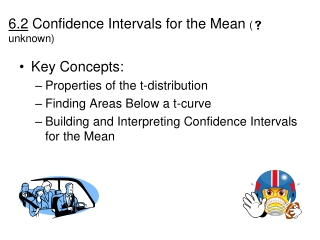DownloadDownload Presentation6.2 Confidence Intervals for the Mean (  unknown )

# 6.2 Confidence Intervals for the Mean (  unknown )

Download Presentation## 6.2 Confidence Intervals for the Mean (  unknown )

- - - - - - - - - - - - - - - - - - - - - - - - - - - E N D - - - - - - - - - - - - - - - - - - - - - - - - - - -
##### Presentation Transcript

1. 6.2 Confidence Intervals for the Mean ( unknown) • Key Concepts: • Properties of the t-distribution • Finding Areas Below a t-curve • Building and Interpreting Confidence Intervals for the Mean

2. 6.2 Confidence Intervals for the Mean ( unknown) • What is the t-distribution? • William S. Gosset • “Studentized” version of the sample mean • If X is normally (or approximately) normally distributed, then the studentized version of the sample mean follows a t-distribution with n-1 degrees of freedom

3. 6.2 Confidence Intervals for the Mean ( unknown) • Properties of the t-distribution • Bell-shaped and symmetric • Shape of the t-curve is determined by the number of degrees of freedom • Total area below every t-curve is 1 • Mean, Median, and Mode are all zero • As the number of degrees of freedom increases, the t-distribution approaches the standard normal distribution

4. 6.2 Confidence Intervals for the Mean ( unknown) • Practice working with t-curves #2, 4 p. 315 • How do we build these confidence intervals? Note: When we construct “t-intervals”, we use the t-curve with n – 1 degrees of freedom.

5. 6.2 Confidence Intervals for the Mean ( unknown) • Practice: #18 p. 315 (Driving Distance to Work) #28 p. 316 (Homework) • How do we know whether a z-interval or a t-interval is more appropriate? • Flowchart on p. 314 will help • More practice: #34 p. 317 (Yards Per Carry) #31 p. 317 (Body Mass Index))